×
Get Full Access to UM - CHM 105 - Study Guide
Get Full Access to UM - CHM 105 - Study Guide

×

UM / Chemistry / CHM 105 / Candle wax is made of?

# Candle wax is made of? Description

##### Description: Hey, I took each lab reading and put it into a simpler study guide. There are definitions, equations, example problems etc for all of the labs. Its very helpful. Enjoy!
18 Pages 221 Views 2 Unlocks
Reviews

CHEM LAB STUDY GUIDE:

## Candle wax is made of?Lab Measurements and Graphing:

Chemical Change: when one or more new substances that differ in  chemical makeup from the original ones are formed.

 Breathing – in comes oxygen and out goes carbon dioxide

Combustion Reaction: the burning of a substance. Need oxygen for  combustion.

 Spark of energy, some oxygen gas, and a combustible material.

Hydrocarbon: a compound that consists of the elements in hydrogen  and carbon. They undergo vigorous reactions and are classified as  combustible materials.

 Candle wax is made of hydrogen and carbon. If you want to learn more check out What is the difference between the abiotic and biotic environment?

-Hydrogen molecules that make up the wax turn into CO2 and  water.

CO2 present if becomes solid.

## What is boiling point?Significant figure rules:

1) All nonzero numbers in a given value are considered significant. 2) Zeros between nonzero numbers are considered significant.  3) Zeros that just hold a place in a given value are not significant.  Ex: 0.003  the three zeros reveal no info.  Don't forget about the age old question of What is added in the reagent hbr?

4) Terminal zeros after a decimal are significant.

Ex: 3.40  0 is significant in this

214.57 + 56.0 = 270.57  must round to 270.6 because smallest SF  after decimal is one (56.0).

Multiplication and Division:

35.689 x 45 = 1606.005  must round to 1600 because smallest  number of SF is 2 in 45.

FALSE

The more precise an intrument, the less amount of sig figs it will have  When making a graph…

## What is the formula to be used when doing the percent error?Include a title, choose appropriate scale for both x and y, include labels for axes, plot points, and include a best fit line or curve. Y=mx+b

Physical Properties Of Inorganic Substances: We also discuss several other topics like Describe loftus’ car crash study
Don't forget about the age old question of How long did thok ding spend in the refugee camp in kenya before he was resettled in the u.s.?

Physical properties: appearance of a metal or an ionic compound. They also can be measured in the lab with solubility, boiling point, and  density.  Don't forget about the age old question of In roper v. simmons (2005), how many juveniles had been executed since 1976?

They involve in no change of chemical makeup of the compound or  element.

Ex: liquid water can be vaporized but its chemical makeup stays the  same. We also discuss several other topics like The father of psychology is who?

 A solid dissolves in a liquid but after the evaporation of water, the  solid remains as it once did before.

What ionic compounds are made up of:

Cations: charged particles (+)

Anions: charged particles (-)

 These can be broken into their corresponding ions.

*Water is polar so it is capable of dissolution. (Polar solvents can  dissolve in ionic compounds).

Less polar = less soluble

*Pure water has a boiling point at 100C at 1 atm

*Water can dissolve MgCl2

Boiling point: occurs when the liquids vapor pressure at is surface  reaches atmospheric pressure. It is pressure dependant. Higher pressure, higher the temp.

AND when a solute is dissolved in pure liquid, the boiling point  increases.

*MT. Everest water boils at 76.5C (has low atm)

Density: mass over volume (g/mL)

MASS: (Container + sample) – (container) = mass of sample VOLUME: graduated cylinder or water displacement

Water displacement: way to measure solid. Fill in water and put the  solid in and then measure the difference. That is the volume of your  solid.

(volume of water + sample) – (volume of water) = volume of sample PERCENT ERROR

Theoretical value – Experimental value

______________________________________________ x 100

Theoretical value

Specific Heat: (cal/gC)

 It takes Lake Michigan until mid July to reach 70F. However the sand  is burning by then. This is due to the specific heat differences.

Specific Heat: of a substance is defined as the amount of heat  energy (cal or joules) needed to raise a gram of the substance 1C or  1K.

(Quantity of heat)

Specific heat = ____________________________________

(Mass)(Temp change)

q(cal)

Specific heat = _________________________

M(g) • ∆T (C)

 q is quantity in cal or joules, m is mass in grams, and ∆T is change in  temperature.

SH with water:

Water possess SH value greater than most other substances. Small amounts of water can absorb a lot of heat which has small  changes in temp.

Large bodies can absorb and store large amounts of heat Large amounts of heat must be lost to decrease the temp of large body of water.

SH of water is more then 9x greater then that of metal -Iron heats a lot faster

-Iron looses heat a lot faster

-Water heats slower

-Water can store heat than an equal mass of iron (and other  liquids).

Calorimeter: an isolated container that can calculate specific heat -Has water and thermometer. Ideally no heat can escape. -Metal is heated and put into the water. Heat flows from an  object so the water temp should change.

-Heat gained by the water = heat lost by the metal.

SH of water is 1000 cal/g  need the mass of water and temp change  of water as well.

EX: a 300g sample of glass at the temperature of 76C was places in a  large container of 1000g of water. The water temperature was initially  23.9C. After the heat transfer it became 27C. Calculate the specific  heat of the glass, and then determine the percent error of the value.

Names and Formulas of Chemical Compounds:

Diatomic elements: two atoms of the element sharing electrons. Hydrogen, Oxygen, Nitrogen, Bromine, Chlorine, Iodine, and  Fluorine.

H2 O2 N2 Br2 Cl2 I2 F2

Compounds: pure substances that are composed of atoms of different elements in a specific ratio.

Molecular compounds: two nonmetal atoms bonded together. Ex: N2O2

Naming: prefixes are used to indicate the number of atoms of  each element involved in the molecular unit.

Mono -1

Di -2

Tri - 3

Tetra - 4

Penta -5

Hexa - 6

Hepta - 7

Octa - 8

Nona - 9

Deca – 10

End of second element has -ide

Ionic compounds: indicate the ratio between the positive ions  (cations) and negative ions (anions) while the molecular formula relays on molecular unit as the smallest entity.

Neutral atom: atoms containing the same amount of protons as  electrons.

The loss of one or more electrons results in a positively charged ion, a  cation.

EX: of element that will loss electron = K+

By loosing one electron Potassium ends up having 8 valence  electrons.

Octet Rule: the main group elements tend to react in a manner that  leaves them with eight valence electrons.

Elements in VA, VIA, VIIA  gain electrons

The suffix –ide is used to naming anions.

Oxidation number: the term used to refer to the charge state  monomic ion.

(Many element can have more than one ionic charge).

Cr2+ Cr3+ Mn2+ Mn3+ Fe2+ Fe3+ Au+ Au3+ Co2+ Co3+ Hg22+ Hg2+ Cu+ Cu2+ Sn2+ Sn4+ Ni2+ Ni3+ Pb2+ Pb4+

Most are transition elements that form common cations.

Polyatomic ions: ions that are made up of one or more element  covalently bonded to another element and often containing oxygen. -If it contains oxyen it will have an –ate

-If this –ate is formed with one or less oxygen the suffix changes  to –ite

*It is important to know the formula and charge of the polyatomic ions.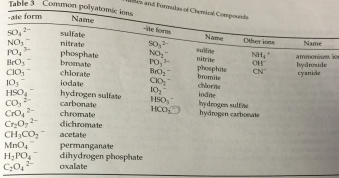Neutral compounds: When ions join with oppositely charged ions in  order to form these compounds.

Formula  the cation is shown or named first, followed by the  anion. The ratio of ions that leads to a neutral compound is termed the formula unit.

Acids: can be identified by its possession of one or more ionizable  hydrogen atoms.

Ionization of the formation of ions from an initially neutral compound.

Binary acid: compounds made up of two elements. First being  Hydrogen and second a nonmetal.

 Naming = hydro + name of the other nonmetal.

Oxyacids: are acids that have oxygen as part of their structure as well as hydrogen and a third nonmetallic element. EX: H2SO4

Polyatomic ion possessing an –ite uses –ous when it converts to be an  acid.

Lewis Dot Structure and Molecular Models:

Chemical bonds: ionic bonds and covalent bonds

Ionic bonds hold ions together.

Covalent bonds: are formed when electrons are shared between  atoms.

Nonpolar covalent bond (pure covalent bond): equal sharing of  electrons.

When 2 different atoms have electronegativity difference of .4 or less  the bon is nonpolar. (sharing is almost equal).

When a bond forms and the electronegativity difference is .4 or more  the bond is polar. (One of the bonds has a stronger attraction than the  other).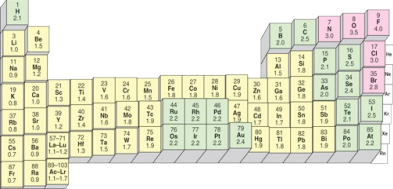Lewis Dot Structure:

1) The outermost electrons are primarily the focus of the atom  when dealing with chemical bonding

2) The octet rule must be followed. (all elements in group A besides  H and He have 8 valence electrons).

3) Electrons surrounding an atom can be shared with another atom or can function as lone pairs.Chemical Reactions and their Classifications:

Chemical equations: are used to describe or represent chemical  reactions (reactants and products).

*chemical names and formulas are required

*The number of each atoms on the left side must equal the  number on the right side.

Symbols: (aq) = compound in water solution, (l) = liquid, (g) = gas, (s)  = solid.

Types:

-Combination reactions: require two or more reactants that result in a  single product, a compound.

2Mg(s) + O2(g)  2MgO (s)

X+ Y  XY

-Decomposition reaction: are opposite of combination reactions. A lot  of the times energy in the form of heat or light is necessary. XY  X + Y

-Substitution reaction: one element in a compound group is replaced  with another element.

2Al(s) + 6HCl(aq)  2AlCl3(aq) + 3H2(aq)

A+ BY  B + AY

-Double displacement reaction: exchange of partners between two  compounds.

Ca(NO3)2(aq) + Na2C2O4(aq)  CaC2O4 + 2NaNO3

AX + BY  AY + BX

***When two salts combine the type of reaction is double displacement as long as chemical reaction occurs.

***When chemical reactions occurs between two substances the  products that for have new identities, new physical and chemical  properties.

Exothermin reaction: a reaction that cuases an increase in heat. Metathesis reaction: a double displacement reaction.

Coefficient: the number placed in front of a part of an equation in  order to balance it.

Electrolytic Solutions and Net Ionic Equations:

Strong electrolyte: a compound that dissociates completely in  solution.

(Strong acids, strong bases, and soluble salts).

They are capable of conducting electricity.

EX: sodium carbonate dissolves readily in water 21.5g/100mL at  20C.

Na2CO3(s)  in water  2Na+(aq) + CO32-(aq)

Weak electrolytes: compounds that do not fully dissociate into their  respective ions.

(Weak acids, weak bases, and partially soluble salts).

EX: silver nitrate dissolves readily .41g/100mL at 25C.

*Amt of H+ is a solution is the basis for its classification as a weak  acid.

Conducting electricity:

Charged anions and cations must be present.

The charged substances must be mobile.

EX: salt, like NaCl is made up of Na+ and Cl-. In the solid state  the ions are not mobile and the compound is a nonconductor. NaCl in  liquid state is able to conduct electricity.

Testing for conductivity: an electrical conductivity apparatus that  consists of two electrodes and a light bulb can be utilized. Cathode: a electrode that accepts the electrons that the electricity  forces.

Due to excess electrons, e-, cations migrate toward the electrode.  Reduction can happen.

Reduction: gain of electrons by a substance.

At the other electrode an electron deficiency is realized. This electrode is termed the anode and anions migrate toward this positively  changed metal.

Anions can transfer electrons to this electrode. The oxidation can take  place.

Oxidation: the loss of electrons by a substance.

If ions are present in the solution the electrical circuit is not complete  and light bulb wont shine.

Glow intensity indicates strength of the electrolytic solution. Strong electrolytes = shine bright

Weak electrolytes = dull glow

Calcium: essential for correct function of some enzymes and is needed  for healthy bones and teeth.

Iron: necessary for the transport of oxygen in the body.  Carbonate and Phosphate: are vital for human existence and are  involved in the correct pH balance of cellular fluids.

*Negative tests does not necessarily mean that the ion is not at all  present. It is hard for the naked eye.

Net ionic equations: simpler equations.

Only the substances that undergo change are involved in the equation.

STEPS:

1) Write the conventional chemical equation and include the states  (l) (g) (s) (aq)

2) Write an ionic equation where the substances that ionize or  dissociate are shown as ions.

a. Do not dissociate solids, liquids, gases, weak acids, weak  bases.

b. Do not dissociate molecular compounds

c. Break down strong acids, strong bases, and soluble ionic  compounds

d. Decompose unstable products

3) Eliminate all the spectator ions those that do not change in the  reaction.

EX:

Chemical equation:

FeCl3(aq) + 3NaOH(aq)  Fe(OH)3(s) + 3NaCl(aq)

Net ionic:

Fe3+(aq) + 3Cl-(aq) + 3Na+(aq) + 3OH-(aq)  Fe(OH)3(s) + 3Na+(aq) +  3Cl-(aq)

Net ionic equation:

Fe3+(aq) + 3OH-(aq)  Fe(OH)3(s)

*There can be times where all of the species in the ionic equation  cancel, then there is no reaction.

Solid state salt = non-electrolytes.

Quiz: T/F

F Aqueous NaCl does not conduct electricity

F Solid NaCl can conduct electricity

F A negative qualitative electrolyte test indicates that the ion is not at  all present in a solution

T strong electrolytes cause electrical conductivity apparatus shine  brightly.

Collection and Measurement of Hydrogen Gas:

Gas = typically a product of a chemical reaction and when it is  produced in a liquid solution, effervescence or bubbling is observed.  Ex: is aqueous acid and carbonate or hydrogen carbonate ion.

Specific gas producing reaction

2CH3CO2H(aq) + Na2CO3(aq)  2NaCH3CO2(aq) + H2CO3(aq) H2CO3(aq)  H2O(l) + CO2(g)

Mg(s) + 2HCl (aq)  MgCl2(aq) + H2(g)

Eudiometer tube: a way to measure the actual volume of hydrogen  gas produced.

It is a graduated glass tube.

You use water displacement.

The tube is filled with small amount of acid and the Mg metal is  secured at the top.

The tube is inverted into a larger glass container, graduated  cylinder.

When the reaction between metal and acid occurs the gas  pushes out the water of the tube into the graduated cylinder.  The volume of gas produced corresponds to the amount of water  that is displaced.

Ideal gas law:

PV = nRT

P = pressure

V = volume

N = number of moles

R = universal gas constant (.0821 L atm/mol K)

T = temperature in K

*Anytime gas is collected over water, some of the water is present as  water vapor which factors in as part of the measured pressure.

Daltons law:

Ptotal = PH2 + PH20

Barometer: room pressure and atmospheric pressure can be read by  this.

Pressure of collected gas = atmospheric pressure.

The water level in eudiometer must = the water level in the grad cyl.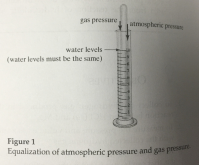*in order for pressures to be equal, the water levels must be at the  same level.

EX: on a given day if the barometric pressure is 754.3 mmHg, the temp is 23C and the volume is 33.2 mL. The H2 pressure and the number of  moles of H2 can be calculated.

Hydrogen gas pressure is the barometric pressure – water vapor  pressure

At 23C, PH20 = 21.1 mmHg. Therefore the pressure is

754.3 mmHg – 21.1 mmHg = 733.2 mmHg

To determine the numbr of moles of H2….

PV = nRT

N = PV/RT

N = (.9647 atm) x (.0332L) / (296 K)(.0821 Lxatm/molxK) = 1.32 x 10-3 mol

Quiz T/F

F Gas producing reaction can occur between reactive metal and an  acid

F The amount of gas produced in the reaction between a reactive  metal and an acid cannot be measured

F Although the gas produced is collected over water the collected gas  does not include anything else besides hydrogen gas. T The volume of gas collected in today’s lab corresponds to the amount of water displaced.

Solution Chemistry on the Aqueous Level:

Aqueous solutions: solid liquid solution when water is the solvent.  All the body fluids are water solutions, ocean, sea, and river  water.

You can describe these solutions by concentration or solubility of  the dissolved substance.

Solvent: substance present in the largest quantity.

Water plays the role of solvent in aqueous solutions.

Solute: substance that dissolves in the solvent.

Solubility: the amount of solute that can dissolve in the solvent.

Dilute solution: solution that contains a relatively small amount of  solute.

Concentrated solution: contains a relatively large amount of solute.

Saturated: a solution containing the maximum amount of the solute  that dissolves in a given amount of solute.

They have reached there solubility limits.

Unsaturated: more solute can be dissolved in a given solvent.

Supersaturated: is not nearly as common as unsaturated and  saturated. It is not stable and holds more than the predicted amount of dissolved solute.

Percent composition: can be used to describe a solution of any type  of solute since the identity or formula mass of the solute is not used. Units: volume/volume%mass/mass%

Volume of solute

_____________________ x 100

Volume of solution

Mass of solute (g)

________________________ x 100

Volume of solution (mL)

Mass of solute

__________________ x 100

Mass of solution

Molarity: the number of moles of solute per liter (M). To find this the  solute must be known.

Molality: the number of moles of solute per kilogram of solvent (m). It reveals the number of molecules or ions of solute per kilogram of  solvent.

EX: 1 m (aq) solution of potassium permanganate contains 1 mole, or  158g og KMnO4

Colligative properties: are properties that are dependant on the  number of amount of solute particles in solution, not the nature or  identity of the solute particles. Have some predicted molarity BUT  have different osmolarity.

Include: freezing point, boiling point, vapor pressure, and osmotic  pressure.

 When salt is added to pot of water, the boiling point increases; the  water boils at a higher temperature.

The solute particles at the surface of the solution function to hold on  the solvent molecules, the H2O molecules.

More particles present the greater the blocking action.

*As amount of solute particles increases, the vapor pressure at the  surface decreases. Overall result is an elevation of the boiling point of  the liquid.

Osmosis: occurs when solvent molecules, or water molecules, pass  through a semi permeable membrane from lower concentration to  higher concentration.

They only allow a certain size of molecules to pass.

When only solvent molecules move through the membrane equilibrium is present.

Osmotic pressure: was number of particles increase, the tendency of the solvent molecules to move to the more concentrated area also  increases.

This causes osmotic pressure.

Solution concentration is human blood = osmolarity of .145M NaCl

Hypertonic: solutions that have a greater concentration than that of a cell.

Cell shrinks because solvent molecules move from the cell to the more  concentrated surroundings.

Hypotonic: Solution possesses a concentration less than that of a cell. The cell bursts. Solvent particles go into the cell.

1 M Al(NO3)3 differ from those of 1 M of fructose.

Salt breaks into four particles as fructose breaks into 4 (Al3+, NO3-, NO3-, NO3-)

Figuring the number of particles in a solution.

Multiple number of particles that result from the dissolution by  concentration.

New units are osmolarity units.

Kb = .5121C/m

Determination of Vitamin C in Behavior:

Absorbic acid: helps prevent scurvy and helps develop tissue. Unused absorbic acid is excreted by the body, making the likelihood of  an overdose far. Prevention of cold and heart disease too.

Vitamin C: is water-soluble. It can be found in citrus fruits, broccoli,  strawberries, lettuce, and peppers.

Considered an antioxidant.

It is unstable and easily oxidized.

 oxidizing agents like DCPIP oxidize with vitamin C.

When DCPIP oxidizes with Vitamin C it becomes reduced.

*Double bonded oxygen and double bonded nitrogen atoms undergo  by a change by bonding to hydrogen atoms.

Reduced DCPIP species is colorless. This is due to oxudation-reduction.

Experiment:

-Vitamin C is added slowly to the DCPIP solution.

-DCPIP is deep blue and turns pink.

-As vitamin C is added oxidation-reduction occurs and some DCPIP is  reduced to its colorless form.

-Pink diminishes and DCPIP is oxidized and color becomes clear.  End point: of titration is when solution becomes clear.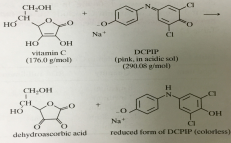n = M x V (moles = Molarity x Volume)

Number of moles DCPIP reduced / volume of DCPIP added to the flask.  M = moles of DCPIP / volume DCPIP, in liters.

Moles of vitamin C are converted to grams by using the molecular  weight. Then grams convert to milligrams.

Quiz:

T the oxygen in the air can readily oxidize vitamin C

T the stiochiometric ratio of vitamin C to DCPIP is 1:1 F Strawberries are not good source of vitamin C

T Vitamin C is known as an absorbic acid

F the lack of Vitamin C will not result in scurvy human body F Vitamin C is not water-soluble

T Vitamin C is very unstable and can be easily oxidized by DCPIP T When DCPIP oxidizes Vitamin C, DCPIP is reduced and Vitamin C is  oxidized.

Problem:

A student standardized a DCPIP solution using .00250 M absorbic acid  solution. Also, 23.1 mL of the abosorbic acid was required in the  titration of 6 mL of DCPIP solution. Calculate the MOLARITY of the  DCPIP solution.

.00250M x .0231L = .00005775/.006L = .009625

Acids, Bases, pH, and Indicators:

Acid: donation of a hydrogen ion, H+ to another substance. Their pH  is lower then 7.

Strong acid: donate essentially all their H+. Nitric acid is an ex. The  more H+ the more acidic.

H2SO4, HCl, HClO4, HBr, and HI

Weak Acid: hydrocynic acid, HCN.

Base: a substance that can accept an H+. Their pH is above 7. OH Groups 1A and IIA metals are considered strong bases. Weak base: ammonia since only small amount of dissolved molecular  ammonia forms in ionized point.

pH = -log[H+]

[H+] = 10-pH

The more hydrogren ions present the smaller the pH becomes. 1x10-2 M has pH of 2.

[H+] = [OH-]

ACID NEUTRAL BASIC

Battery acid apples milk human blood baking  soda ammonia

Indicator: a way of measuring the pH of a solution. They change color with change in pH.

Indicators act as weak acids or weak bases.

pH paper: paper strips coated with indicators.

pH meter: instrument equipped with electrodes and gives a direct and accurate reading of pH.

Concentration of H+ increases, pH value decreases.

pH has not units.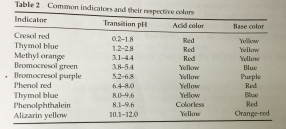Page ExpiredIt looks like your free minutes have expired! Lucky for you we have all the content you need, just sign up here